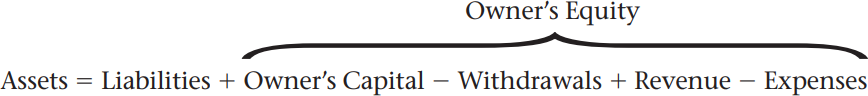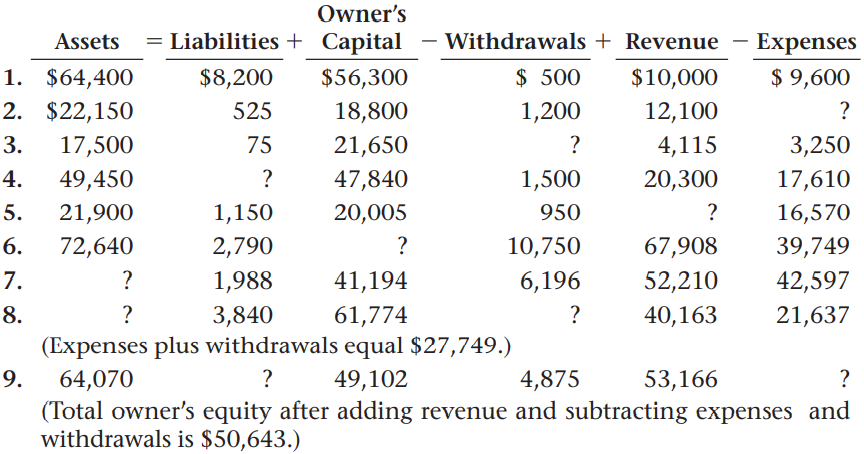×

×

# Completing the Accounting Equation With the addition of temporary accounts, the basicISBN: 9780078688294 416

## Solution for problem 5-8 Chapter 5

Accounting: First Year Course | 1st Edition

• Textbook Solutions
• 2901 Step-by-step solutions solved by professors and subject experts
• Get 24/7 help from StudySoup virtual teaching assistantsAccounting: First Year Course | 1st Edition

4 5 1 283 Reviews
21
3
Problem 5-8

Completing the Accounting Equation With the addition of temporary accounts, the basic accounting equation can be expressed as follows: Owners EquityAssets Liabilities Owners Capital Withdrawals Revenue Expenses Instructions Using the expanded equation shown above, determine the missing amounts for the following accounting equations. Use the form in your working papers. The first equation is completed as an example.Assets LiabilitiesOwners Capital Withdrawals Revenue Expenses 1. $64,400$8,200 $56,300$ 500 $10,000$ 9,600 2. $22,150 525 18,800 1,200 12,100 ? 3. 17,500 75 21,650 ? 4,115 3,250 4. 49,450 ? 47,840 1,500 20,300 17,610 5. 21,900 1,150 20,005 950 ? 16,570 6. 72,640 2,790 ? 10,750 67,908 39,749 7. ? 1,988 41,194 6,196 52,210 42,597 8. ? 3,840 61,774 ? 40,163 21,637 (Expenses plus withdrawals equal$27,749.) 9. 64,070 ? 49,102 4,875 53,166 ? (Total owners equity after adding revenue and subtracting expenses and withdrawals is $50,643.) Step-by-Step Solution: Problem 5.8 Completing the Accounting Equation With the addition of temporary accounts, the basic accounting equation can be expressed as follows:Instructions Using the expanded equation shown above, determine the missing amounts for the following accounting equations. Use the form in your working papers. The first equation is completed as an example.For equation 7, calculate the sum of owner’s equity. Step by Step Solution Step 1 of 3 Accounting equation refers to a foundation set for building up the double entry system which states that the sum of liabilities and owners’ equity is equal to the total of assets owned by the organization. Step 2 of 3 ###### Chapter 5, Problem 5-8 is Solved Step 3 of 3 ##### Textbook: Accounting: First Year Course ##### Edition: 1 ##### Author: Glencoe ##### ISBN: 9780078688294 This textbook survival guide was created for the textbook: Accounting: First Year Course, edition: 1. Since the solution to 5-8 from 5 chapter was answered, more than 1598 students have viewed the full step-by-step answer. The full step-by-step solution to problem: 5-8 from chapter: 5 was answered by , our top Business solution expert on 03/14/18, 05:31PM. Accounting: First Year Course was written by and is associated to the ISBN: 9780078688294. The answer to “Completing the Accounting Equation With the addition of temporary accounts, the basic accounting equation can be expressed as follows: Owners EquityAssets Liabilities Owners Capital Withdrawals Revenue Expenses Instructions Using the expanded equation shown above, determine the missing amounts for the following accounting equations. Use the form in your working papers. The first equation is completed as an example.Assets LiabilitiesOwners Capital Withdrawals Revenue Expenses 1.$64,400 $8,200$56,300 $500$10,000 $9,600 2.$22,150 525 18,800 1,200 12,100 ? 3. 17,500 75 21,650 ? 4,115 3,250 4. 49,450 ? 47,840 1,500 20,300 17,610 5. 21,900 1,150 20,005 950 ? 16,570 6. 72,640 2,790 ? 10,750 67,908 39,749 7. ? 1,988 41,194 6,196 52,210 42,597 8. ? 3,840 61,774 ? 40,163 21,637 (Expenses plus withdrawals equal $27,749.) 9. 64,070 ? 49,102 4,875 53,166 ? (Total owners equity after adding revenue and subtracting expenses and withdrawals is$50,643.)” is broken down into a number of easy to follow steps, and 146 words. This full solution covers the following key subjects: . This expansive textbook survival guide covers 29 chapters, and 429 solutions.

Unlock Textbook Solution